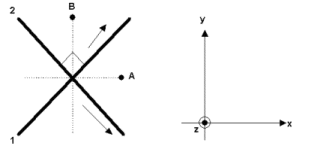Teaching Physics with the Physics Suite Edward F. Redish

Magnetic forces

In the figure at the left below are shown parts of two long current carrying wires labeled 1 and 2. The wires lie in the same plane and cross at right angles at the point indicated. When it carries a current, each wire carries the same amount of current in the direction shown. At the right is shown a set of coordinate directions for describing the direction of vectors.For each of the vectors labeled (a)-(c) below indicate the direction of the vector by specifying its direction using the coordinate system shown. For example, you might specify "the +x direction" or "the -z direction" or "in the x-y plane at 45ş between the +x and +y directions." If the magnitude of the vector requested is zero, write "0".

1. The direction of the force on a positively charged ion at the point B moving in the +y direction if only wire 1 carries current.
2. The direction of the force on a positively charged ion at the point B moving in the -z direction if both wires carry current.
3. The direction of the force on a positively charged ion at the point A moving in the +x direction if only wire 2 carries current.
For the next two parts of the problem, select which answer is correct if both wires carry current.
1. The magnetic force on wire 1 will
1. push it in the -z direction
2. push it in the +z direction
3. tend to rotate it clockwise about the joining point
4. tend to rotate it counter-clockwise about the joining point
5. none of the above.
2. The magnetic force on wire 2 will
1. push it in the -z direction
2. push it in the +z direction
3. tend to rotate it clockwise about the joining point
4. tend to rotate it counter-clockwise about the joining point
5. none of the above.

Not finding what you wanted? Check the Site Map for more information.